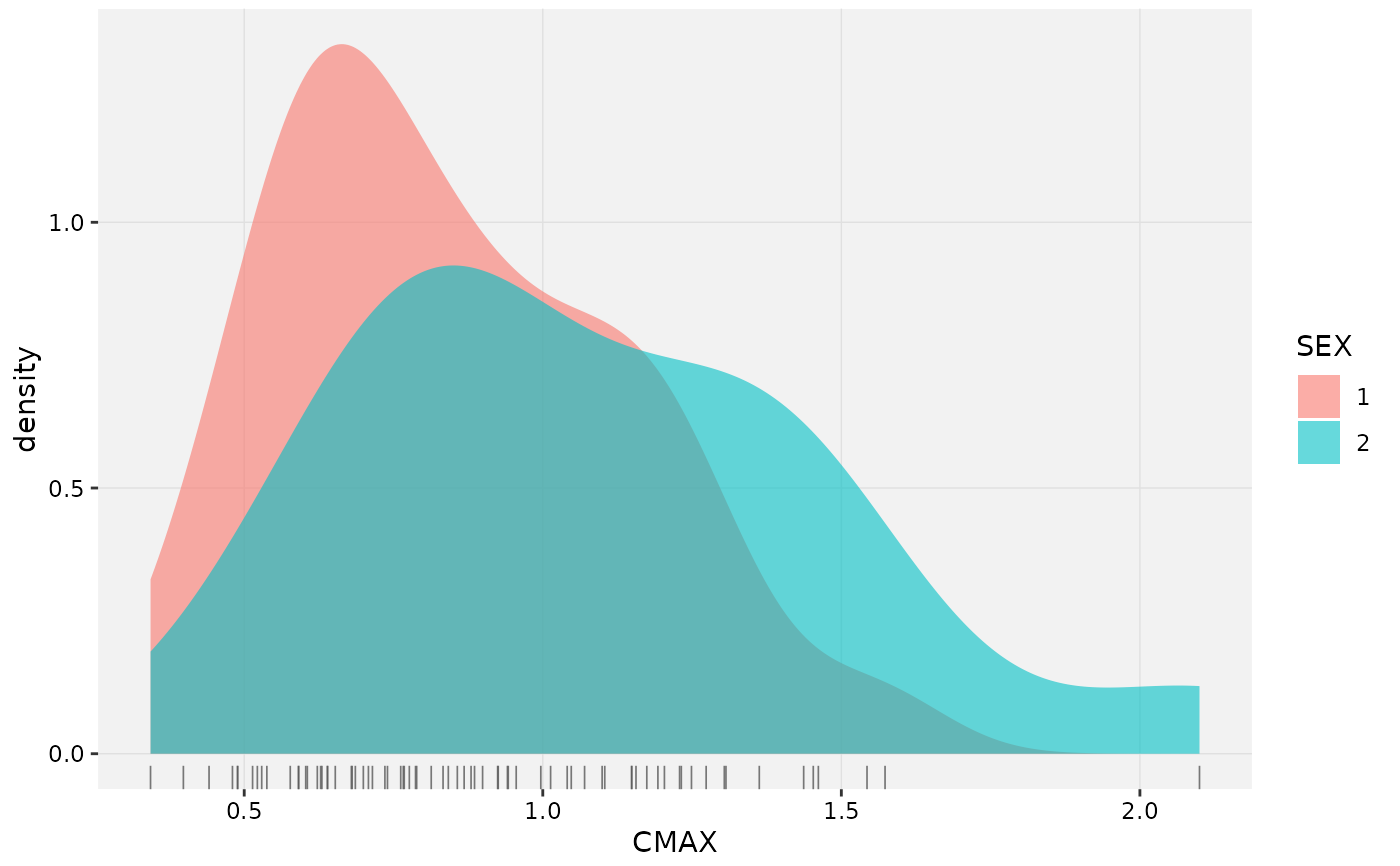group_by() takes an existing table and converts it into a grouped table where operations are performed "by group". ungroup() removes grouping. summarize() reduces multiple values down to a single value.

# S3 method for xpose_data
group_by(.data, ..., .problem, .source, .where)

# S3 method for xpose_data
ungroup(x, ..., .problem, .source, .where)

# S3 method for xpose_data
summarise(.data, ..., .problem, .source, .where)

# S3 method for xpose_data
summarize(.data, ..., .problem, .source, .where)

## Arguments

.data An xpose database object. Name-value pairs of expressions. Use NULL to drop a variable. These arguments are automatically quoted and evaluated in the context of the data frame. They support unquoting and splicing. See the dplyr vignette("programming") for an introduction to these concepts. The problem from which the data will be modified The source of the data in the xpdb. Can either be 'data' or an output file extension e.g. 'phi'. A vector of element names to be edited in special (e.g. .where = c('vpc_dat', 'aggr_obs') with vpc). Same as .data (used for consistency with dplyr functions).

## Examples

# Create a distribution plot of Cmax
xpdb_ex_pk %>%
group_by(ID, SEX, .problem = 1) %>%
summarize(CMAX = max(DV), .problem = 1) %>%
ungroup(.problem = 1) %>%
xplot_distrib(aes(x = CMAX, density_fill = SEX), type = 'dr')#> Using data from \$prob no.1